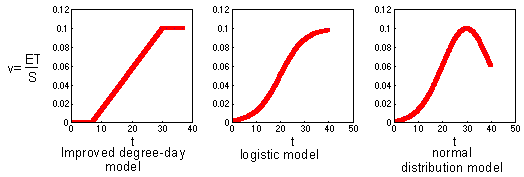## 8.5. Other non-linear models of development

### Other non-linear models of development

Improved degree-day model inherited a linear relationship between development rate and temperature (between two temperature limits tmin and tmax) from the simple degree-day model. Now it is time to make the next logical step: not to use linear relationships at all. Below two alternative models are shown: the logistic model and the normal distribution:Non-linear models like the logistic model or the normal distribution are easy to interpret for experiments with constant temperature. In this case, development rate can be defined as a reciprocal of development time (v = 1/T). But what is development rate if temperature changes? We cannot accumulate degree-days any more because the model is non-linear. In attempt to answer this question a new concept has been developed: a concept of physiological time.

Alexei Sharov 12/5/98